# Grant's(?) Rule

Consider a characteristic equation (denominator of the closed-loop transfer function) of the form

P(s) = (s+a)(s+b)(s+c)(s+d) = 0.

If we multiply out this characteristic equation, we get

P(s) = s4 + ws3 + xs2 + ys + z = 0.

Clearly, the coefficient w is the sum of all the pole locations.

w = a+b+c+d

Therefore, if we have a characteristic equation written in terms of the loop transfer function as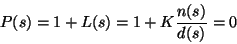P(s) = d(s)+Kn(s) = 0

and we multiply out the left side, and the order of d(s) is two or more greater than the order of n(s), if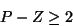then the coefficient of sP-1 is independent of K. In other words, the sum of all the roots (closed-loop poles) is constant and equal to the sum of the open-loop poles. This result gives us our seventh root-locus rule:

Rule 7
If there are two or more excess poles than zeros (), then for any gain K, the sum of the real parts of the closed-loop poles (or the average distance from the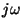-axis) is constant.

For example, compare the root-locus plots in Figures 3 and 4 for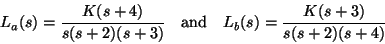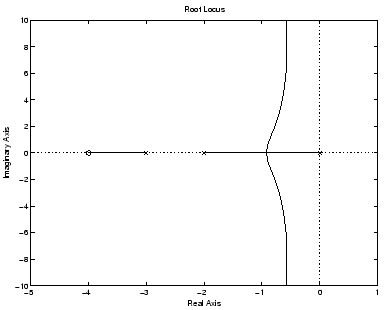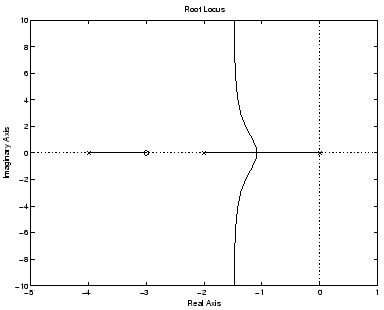In Figure 3, since the high-frequency pole is moving left along the real axis, the complex-conjugate poles have to move to the right. In Figure 4, since the high-frequency pole is moving right along the real axis, the complex-conjugate poles have to move to the left. We could have also found this result by considering the centroid of the asymptotes. For Figure 3, the centroid is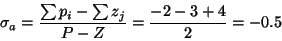and for Figure 4 the centroid is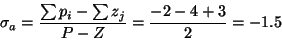## The Question

I have heard this fact called Grant's Rule.'' However, it is not listed as such in any of the primary root locus books by Walter Evans  , John Truxal , or even Chestnut and Mayer .

The only reference that I've been able to find that names this fact Grant's Rule'' is Linear Control System Analysis and Design by D'Azzo and Houpis . They reference an unpublished paper (from North American Aviation) by A. J. Grant  entitled The conservation of the sum of the system roots as applied to the root locus method,'' dated April 10, 1953 . I have tried in vain to find this paper. I have written Professors D'Azzo and Houpis, and even the company historian at Boeing (Boeing now owns North American Aviation) without success.

If you have a copy of this paper that you can send me, or knowledge of other texts or references that label this fact Grant's Rule,'' I would greatly appreciate hearing from you.

Kent H Lundberg
2002-11-14